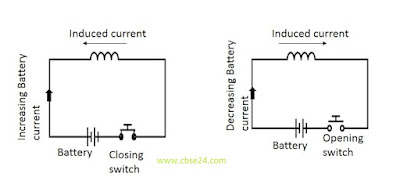# Self-Induction | www.cbse24.com

Self-induction:-When a current flows in a coil, it gives rise to magnetic flux in a coil itself. As the strength of current changes, the linked magnetic flux changes, and an opposite emf is induced in the coil. This emf is called Self-induced emf or back emf and the phenomenon is known as self-induction.

Self-induction is the phenomenon of production of induced emf in a coil when a changing current posses through it.

In figure shows a battery and a switch connected in series to a coil. As the switch is closed, the current increases and hence the magnetic flux through the coil increase from zero to a magnetic flux through the coil increases from zero to a maximum value and the induced current flows in the opposite direction of the battery current as the switch is opened the current and hence the magnetic flux through the coil decrease from a maximum value to zero and the induced current flows in the same direction as that of the batteryThe induced current in a coil when the circuit is(1) closed (2) opened

## Coefficient of self - induction:

At any instant, the magnetic flux Φ linked with a coil is proportional to the current I through it

ΦI

or  ϕ=LI.................(1)

Where L is a constant for the given coil and is called self-inductance or more often simply inductance. It is also called the coefficient of self-inductance of the coil. Any change in current sets up an induced emf in the coil given by

e=dϕdt=LdI.............(2)dt

If in equation (1) I=1 then  ϕ=L
Thus the self-inductance of a coil is numerically equal to the magnetic flux linked with the coil when a unit current flows through it.

Again from equation (2) if  dIdt=then,e=L

Thus the self-inductance of a coil may be defined as the induced emf set up in the coil due to a unit rate of change of current through it.

## Units of self-inductance:-

L=edIdt

L=1V1As1=VsA1=1henery(H)

The self-inductance of a coil is said to be one henry if an induced emf of one volt is set up in it when the current in it changes at the rate of one ampere per second.

1henry(H)=1VsA1=1WbA1

*